# Assignments

The central theme of the following assignments is to calculate the derivative of an implicitly defined function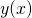.

1. Calculate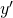if: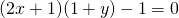Solution

2. Calculateif: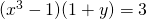Solution

3. Calculateif: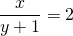Solution

4. Calculateif:Solution

5. Calculate the tangent line at the point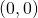of the graph of the following expression: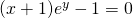Solution

6. Calculate the tangent line at the point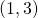of the graph of the following expression: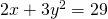Solution

7. Calculate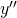if: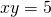Solution

8. If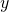is a differentiable function ofsatisfying the following equation: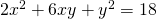calculate.

Solution

9. Calculate the tangent line at the pointof the graph of the following expression: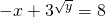Solution

10. The equation:definesas a differentiable function ofclose to the point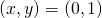.

Calculate the tangent line of the graph at this point.

Solution

0